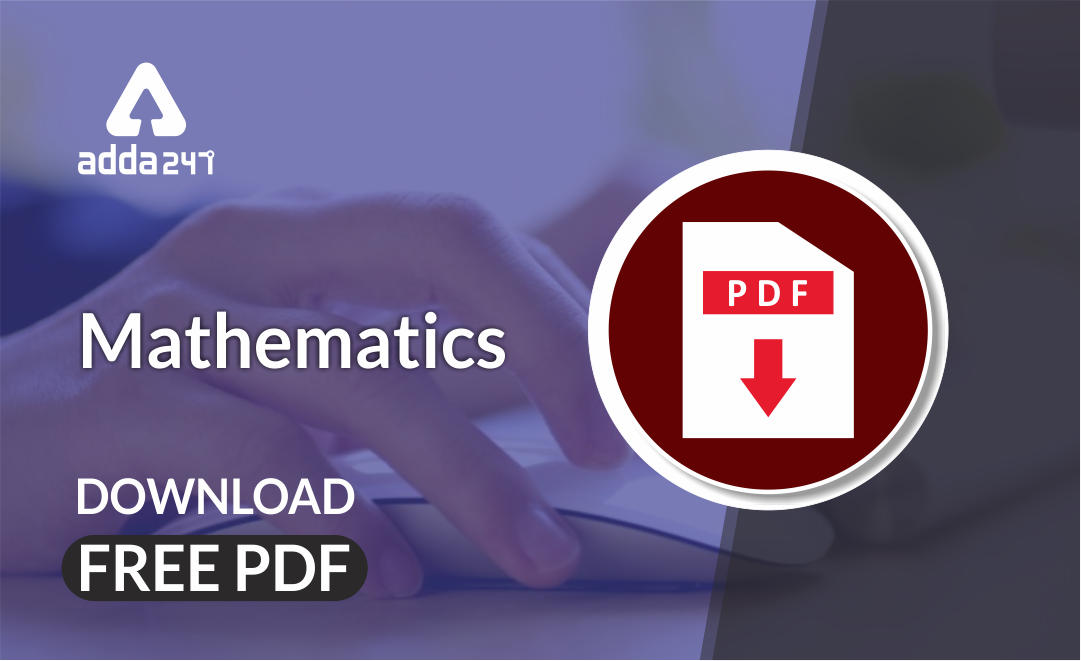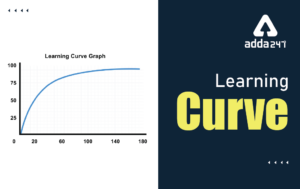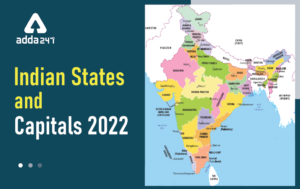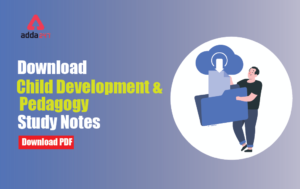Latest Teaching jobs   »   Aims And Objectives Of Teaching Mathematics...

# Aims And Objectives Of Teaching Mathematics Notes For All Teaching ExamsMathematics is an equally important section for CTET, MPTET, KVS & DSSSB Exams and has even more abundant importance in some other exams conducted by central or state govt. Generally, there are questions asked related to basic concepts and Facts of the Aim and objectives of the teaching Mathematics.

To let you make the most of Mathematics section, we are providing important facts related to the Mathematics Pedagogy. At least 10-15 questions are asked from this topic in most of the teaching exams. We wish you all the best of luck to come over the fear of the Mathematics section.

How to Overcome Exam Fever, Especially When You Fear Maths

## AIMS AND OBJECTIVES OF TEACHING MATHEMATICS

Mathematics: The abstract science which investigates deductively the conclusions implicit in the elementary conceptions of spatial and numerical relations, and which includes as its main divisions, geometry, arithmetic, and algebra.

According to Kant “Mathematics is the indispensable instrument of all physical researches”. Gauss stated “Mathematics is the queen of sciences and arithmetic is queen of all mathematics”. Bacon said “Mathematics is the gateway and key to all sciences”.

According to Lindsay, “Mathematics is the language of physical sciences and certainly no more marvellous language was created by the mind of man”.

Mathematics is commonly defined as the study of patterns of structure, chance, and space; more informally, one might say it is the study of figures and numbers. In the formalist view, it is the investigation of axiomatically defined abstract structures using logic and mathematical notation; other views are described in philosophy of mathematics.

### Mathematics and Its Relationship with Other Subjects

Mathematics with arts: The arts and mathematics involve students understanding of relationships between time and space, rhythm and line through the experience of these abstract concepts in various arts forms and mathematical ideas.

Mathematics with civics and citizenship: The concepts developed in the study of mathematics are applicable to a range of civic and citizenship understandings. Mathematical structure and working play essential roles in key aspects of our society as well as key civics concepts.

Mathematics in Geography: Geography is nothing but a scientific and mathematical description of our earth in its universe. The dimension and magnitude of earth, its situation and position in the universe the formation of days and nights, lunar and solar eclipses, latitude and longitude, maximum and minimum rainfall, etc are some of the numerous learning areas of geography which need the application of mathematics.

Mathematics with communication: Developments of the languages of mathematics are crucial to its practical application. Students learn to use the language and concepts of mathematics both within the discipline itself and also its applications to modelling and problem solving across the other domains.

Mathematics with English: Mathematics, including the use of conjectures and proof, has clear links to the development of structures and coherent argument in speaking writing.

Mathematics with health and physical education: In health and physical education, mathematics provides tools and procedures which can be used to model situations and solve problems in areas such as:

1. Scoring different sporting events involving time distance, weight and number as variables.
2. Calculating percentage improvement in results from data collected through fitness testing or performance in physical activities.

Mathematics with history: The study of history includes the analysis and interpretation of a range of historical information including population charts and diagrams and other statistical information.

Mathematics with science: In science students use measurement and number concepts particularly in data collection estimation of error analysis and modes of reporting. The mathematics domain supports students in developing number handling skills.

### Need and Importance of Objective–Based Teaching of Mathematics

• Teaching and learning of basic numeracy skills to all pupils.
• Teaching of practical mathematics (arithmetic, elementary algebra, plane and solid geometry, trigonometry) to most pupils, to equip them to follow a trade or craft.
• Teaching of abstract mathematical concepts (such as set and function) at an early age
• Teaching of selected areas of mathematics (such as Euclidean geometry) as an example of an axiomatic system and a model of deductive reasoning

### Aims of Teaching Mathematics

Develop a good understanding of numbers and the number system

1. Maximising their counting ability.
2. Achieving a sound grasp of the properties of numbers and number sequences including negative numbers.
3. Achieving a good understanding of place value and ordering, including reading and writing numbers.
4. Understanding the principles and practice of estimating rounding.

Improving speed

1. Achieving a good understanding of number operations and relationships.
2. Achieving rapid mental recall of numbers facts
3. Maximizing the ability to undertake calculation using pencil and paper methods.

To develop a good ability to solve problem.

1. Developing the ability to make decisions. E.g. deciding which operation and method of calculation to use.
2. Improving the ability to solve problems involving numbers in context.

The Three Domains of Learning:

Cognitive: mental skills (knowledge)

Affective: growth in feelings or emotional areas (attitude or self)

Psychomotor: manual or physical skills (skills)

Objectives should be SMART:

Specific: The teacher should state clearly what the student should know/be able to do, and at what level.

Measurable: The teacher must be able to conceive of how their attainment might be assessed

Attainable: by the students

Realistic: Could be seen as similar to attainability, but refers to their appropriateness to the

Time: appropriate or achievable within the time-span of the session/lesson/course

Check More Mathematics Study Notes Here

### GENERAL INSTRUCTIONAL OBJECTIVES

An instructional objective is an intended outcome of instruction that has been stated in appropriate general terms to encompass a domain of student performance. It must be further defined by a set of specific learning outcomes. Objectives can be helpful in instructional planning, during the teaching/learning process, and when assessing student progress. Instructional objectives are often either ignored (by both teachers and students) or are, at best, occasionally referred to.

Knowledge: the pupil acquires knowledge of terms, concepts, symbols, definitions, principles, processes and formulae of mathematics at the secondary stage.

Specification: To demonstrate the achievement of above objectives, the pupil

1. Recalls or reproduces
2. Recognizes Understanding: the pupil develops understanding of terms, concepts, symbols, definitions, principles, processes, and formulae of mathematics at the secondary stage.

Application: the pupil applies his knowledge and understanding of mathematics to unfamiliar situation.

Specifications: The pupil, 1. Analyses and finds out what is required.

1. Finds out the adequacy, superfluity or relevancy of data
2. Establishes relationship among the data
3. Suggests alternative methods
4. Generalizes
5. Infers

Skill: to acquire skills of computation, drawing geometrical figures and grapes reaching tables, charts, graphs etc.

The pupil acquires skill in

1. Drawing geometrical figures and graphs
2. Reading tables, charts, graphs etc.

Specifications: The pupil,

1. Carries out oral calculations with ease and speed.
2. Carries out written calculations with ease and speed.

•Learning Curve - What is Learning Curve?...
•रस - परिभाषा, भे�...
•पठन कौशल- परिभा�...
•अलंकार - परिभाष�...
•Indian States and Capitals 2022: 28 Stat...
•New Education Policy(NEP) PDF - नई �...